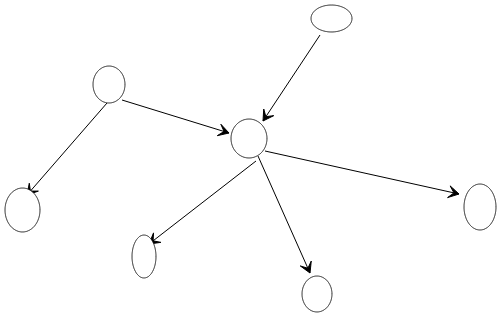# Page Rank

Goal: rank the search result.

“Authority” of documents: Number of citation.

So we create a Directional Graph.# Authority to page

1. $$X_i\in R$$.
2. $$X_i=\sum_{j\in Parent(i)}\frac{1}{N}X_j$$.
3. Default visibility 1.

Combine the 2 and 3, we have: $$X = dWX + (1-d)1$$.

# Solution to this equation

1. Stupid way, take inverse and solve.
2. Contraction Function iteration.

# Fix Point and Dynamic System

For $$F(X)=X=dWX+(1-d)1$$, find $$X_*: X_*=F(X_*)$$.

So how to find $$X_*$$?

1. Lipshiz Continuity: For any $$X_1, X_2 \in X$$, $$\|G(X_1)-G(X_2)\|\leq\varrho\|X_1-X_2\|$$, which $$0<\varrho<1$$.

2. Repeatedly apply $$G$$ to $$G^{n-1}(X)$$ to get $$G^n(X)$$: $$\|G^n(X)-G^n(Y)\|\leq \varrho^n d$$.

Now we prove any initial $$X$$ will end up in the same fix point after repeatedly apply $$G$$.

# Maximize The Visibility of a Page Community

## Page Community

A group of pages, connect with each other. The way to maximize its visibility is to maximize its energy.

$E_G=|G|+E^{Into}_G-E^{Out}_G-E^{dp}_G$

where $$\|G\|$$ is the number of page in the community.

1. $E^{Into}_G=\frac{1-d}{d}\sum_{p\in Into(G)}x_p\rho_p$
2. $E^{Out}_G=\frac{1-d}{d}\sum_{p\in Out(G)}x_p(1-\rho_p)$
3. $E^{dp}_G=\frac{1-d}{d}\sum_{p\in dp(G)}x_p$
4. $$dp$$ means the pages which have no outer link.

5. $$\rho_p$$ means the fraction of its outgoing links that stay inside $$G$$.